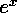<b>double exp(double x)</b>

Document: The C Standard Librarydouble log(double x)Mathematical Functions: math.hdouble atan2(double ydouble x)

## double exp(double x)

The return value is the exponential function of x, i.e..

McMullin@eeng.dcu.ie
Fri Mar 29 14:35:38 GMT 1996